# BINARY TREE

Binary tree is a set of finite nodes which is either empty or consists of one or more nodes in which each node has at most two disjoint binary subtrees called left subtree or right subtree respectively. Thus we can say that there may be a zero degree node or a one degree node or a two degree node.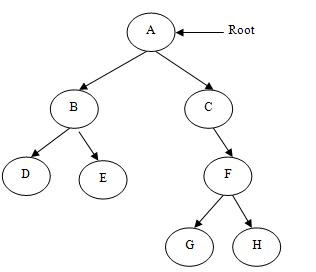Figure-(a) shows a typical binary tree.

In figure-(a)  A is the root node, B and C are the left subtree and right subtree of root node A respectively. This binary tree is not a strictly binary tree. A binary tree is strictly binary tree if every non-terminal node has two subtrees or you can say if every non-terminal node consists of non-empty left subtree as well as non-empty right subtree.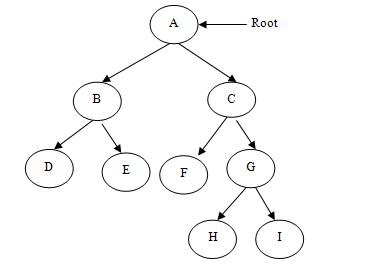Figure-(b) shows a strictly binary tree.Figure (c)

The binary trees that we have discussed so far are not complete binary trees. A complete binary tree is as shown in figure-(c). Thus in  complete binary tree if there are ‘n’ number of nodes at level ‘m’ then it contains at most ‘2n’ nodes at level ‘m+1’.

### Implementation of Binary Trees

Binary trees are implemented in two ways:

1. Array implementation of Binary Trees
2. Linked List implementation of Binary Trees

### Array implementation of Binary Trees

In an array implementation of binary trees we use one-dimensional array. The nodes stored in an array are accessible sequentially. Consider the complete binary tree. and number the nodes beginning with the root from left to right at each level. The root node is numbered 0, then the left child as 1 and right child as 2 and so on.

Now if we place node number at index 0 then in successive positions the left child and right child are stored. Figure-(d) representation of this binary tree.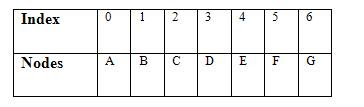Figure (d)

This table concludes that if father is at index ‘n’ then its left children will be at index 2n+1 and its right children will be at index 2n+2. Let we want to find out the left and right child position of node C. Since the node C is placed at index 2, therefore its left child would be at index 5 (2*2+1) and its right child would be at index 6 (2*2+2).

The situation is pleasant so far because it is complete binary tree. But if the binary tree is not complete then it definitely results in unnecessary wastage of memory space because array is static in nature.  For example let we have a binary tree as shown in figure- (e).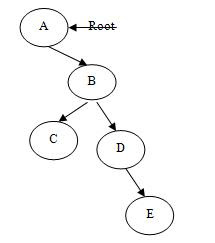figure (e)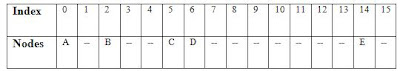The array representation of this binary tree is as shown in figure- (f)

From this table it is clear that if a tree is far from its complete behavior then the array implementation would waste a lot of memory space. This type of problem is overcome by implementing the binary tree in linked list.

### Linked List implementation of Binary Trees

In this we will use a linked list in which elements are treated as nodes. Each node a binary tree has three fields, as follows:

• Left child
• Data
• Right child

The data field contains the value of the node, the left child contains the information about the left child and right child contains the information about the right child. Figure-(g) shows this.The structure of a binary node is defined as:

```struct treenode
{
struct treenode *lchild;
int data;
struct treenode *rchild;
};
typedef struct treenode BTNode;```

Figure-(h)  shows the linked list representation of a binary tree shown in figure-(e)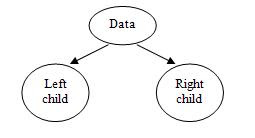Firgure (h)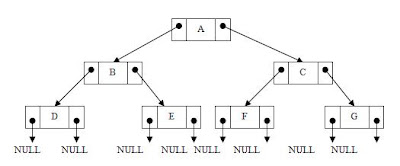From the figure it is clear that the left link field and right link field of leaf nodes contains a NULL value each. However if a parent node has empty left child then its left link field would be set to NULL and if it has empty right child then its right link field is set to NULL.